# Hitachi Content Platform​### Need to convert date from full date (locale=en_US.UTF-8) to ISO

• Object Storage
• Hitachi Content Intelligence HCI
posted 09-12-2018 11:35

Hi,

I have a problem converting a date to ISO format in the Date Conversion stage. There are two possible formats for the date:

Fri, 2 Feb 2018 07:57:34 +0100

Fri, 2 Feb 2018 07:57:34 +0100 (CET)

I have modified the standard Date Conversion stage, added my field name, and:

- regular expression:

^\w{3},\s\d{1,2}\s\w{3}\s\d{4}\s\d{1,2}:\d{1,2}:\d{1,2}\s[\+\-]\d{4}

I think this is correct, except for probably the comma (should it be escaped? how? standard Perl/Tcl or Vim don't offer a way to escape it, except possibly \b for word boundaries).

- scan pattern:

EEE, dd MMM yyyy HH:mm:ss X

To the best of my knowledge, this is okay right up until the X, where I have no idea what to put. I'd appreciate input on this as well.

Marek

#HitachiContentIntelligenceHCI

You are just missing \s\(\w{3}\) at the end, to match the timezone.

https://www.regextester.com/?fam=104922

to capture this in a more generic way whether you have the (...) or not use this expression:

^\w{3},\s\d{1,2}\s\w{3}\s\d{4}\s\d{1,2}:\d{1,2}:\d{1,2}\s[\+\-]\d{4}(?:\s\(\w+\))?

This scan pattern would work: EEE, dd MMM yyyy HH:mm:ssZ

Let me retrace the steps I did to debug this.

I created a Filter step to rename the field I'm working on, and it recognizes the field and renames it, so that's not it.

To clarify, I put the scan format in there:

Regular Expression: ^\w{3}\,\s\d{1,2}\s\w{3}\s\d{4}\s\d{1,2}:\d{1,2}:\d{1,2}\s[\+\-]\d{4}(?:\s\(\w+\))?

Scan Pattern: EEE, dd MMM yyyy HH:mm:ssZ

I also tried a simplified regex:

^\w{3}\,\s\d{1,2}\s\w{3}\s\d{4}\s\d{1,2}:\d{1,2}:\d{1,2}

and scan pattern:

EEE, dd MMM yyyy HH:mm:ss

HCI still fails to recognize it.

I then tried the first format with several different scan patterns:

Scan Pattern: EEE, dd MMM yyyy HH:mm:ss Z

Scan Pattern: EEE, dd MMM yyyy HH:mm:ss(Z)

Scan Pattern: EEE, dd MMM yyyy HH:mm:ss (Z)

Still didn't work. I realized that I'm using a 1-31 format for days (instead of 01-31), so I retried with this scan pattern:

EEE, d MMM yyyy HH:mm:ssZ

Still didn't work. I realized that it's probably a first match, so I removed all duplicate regular expressions and retried with just one item. Still nothing.

Just to see if this step is actually reading the field, I put the date into two other fields of custom metadata and formatted them differently: One to yyyy-MM-dd'T'HH:mm:ssZ and another by translating the weekday and month names to my local language (just in case HCI is configured in locale pl_PL). The first one was recognized and transformed (so the step does read the custom metadata field), the second one was still ignored (so luckily, it's not about the locale).

Finally, just to rule out the possibility that the step is not doing anything for whatever reason, I added a step to transform that above format (yyyy-MM-dd'T'HH:mm:ssZ), and it worked.

The last thing I did was, I removed the literal time zone representation, from this:

Fri, 2 Feb 2018 07:57:34 +0100 (CET)

to this:

Fri, 2 Feb 2018 07:57:34 +0100

That one finally worked. However, I have 1.5 million objects to upload with associated metadata as of now, and some of them have the time zone name and some of them don't. Is there any way to make sure that both formats are recognized?

What is functionally different between "+0100" and "+0100 (CET)" that a catch-all scan pattern recognized the former, but not the latter? I don't know, should I make two separate scan formats, with one that has:

^\w{3},\s\d{1,2}\s\w{3}\s\d{4}\s\d{1,2}:\d{1,2}:\d{1,2}\s[\+\-]\d{4}\$

EEE, d MMM yyyy HH:mm:ss X

And this one:

^\w{3},\s\d{1,2}\s\w{3}\s\d{4}\s\d{1,2}:\d{1,2}:\d{1,2}\s[\+\-]\d{4}(\s\(\w+\))\$

EEE, d MMM yyyy HH:mm:ss X (X)

Would that actually work (with two "X" references)?

I assume you added the metadata field name in the date conversion stage?

The last reg expression will capture the date pattern you have. But, do you have a sample object data you can share?... I can run it through one of my HCI instances here.

Hi Dia,

There are two timestamps, the first one worked, the second one didn't:

Tue, 2 Jan 2018 19:03:52 +0100

Tue, 2 Jan 2018 19:03:52 +0100 (CET)

I finally solved the problem. It was in using a non-capturing group instead of positive lookahead. In fact, the online documentation uses the correct syntax, but the incorrect semantics.

To quote the documentation:

Tip:  You can use regular expressions with non-capturing groups [sic!] [emphasis mine] to omit irrelevant characters from field values.  For example, in this date value, the digits 123 are not part of the date and should be excluded from conversion:

19570110_220434.123

To omit the invalid digits, use a regular expression that selects only the date portion. For example:

\d{8}_\d{6}(?=\.\d{3})

The corresponding date format for this expression is:

yyyyMMdd_HHmmss

The "?=" quantifier is positive lookahead, while "?:" is non-capturing group. Here's the regular expression that captures the timestamp correctly:

^\w{3},\s\d{1,2}\s\w{3}\s\d{4}\s\d{1,2}:\d{1,2}:\d{1,2}\s[\+\-]\d{4}(?=\s\(\w+\))

This should also work, but I don't want to test my luck:

^\w{3},\s\d{1,2}\s\w{3}\s\d{4}\s\d{1,2}:\d{1,2}:\d{1,2}\s[\+\-]\d{4}(?=\s\(\w+\)\$)

Note that these will not work:

^\w{3},\s\d{1,2}\s\w{3}\s\d{4}\s\d{1,2}:\d{1,2}:\d{1,2}\s[\+\-]\d{4}(?=\s\(\w+\))\$

^\w{3},\s\d{1,2}\s\w{3}\s\d{4}\s\d{1,2}:\d{1,2}:\d{1,2}\s[\+\-]\d{4}(?:\s\(\w+\))

For now it looks like the case is closed. Thanks for putting me on the right track.

As an aside, is there a way to get HCI to convert a date that does not explicitly include the time offset, like these:

Tue, 2 Jan 2018 19:03:52 (CET)

Tue, 2 Jan 2018 19:03:52 CET

This is a just-in-case question, in case I run into it.

Great you were able to find the right pattern and thank you for sharing. It's interesting it did not work. The pattern I sent with ?: is a non-capturing group and with "?" at the end it should match 0 or 1 instances of the timezone.

Not sure what happened on your HCI instance, but it is working for you now, so it won't matter which pattern you use

This one worked okay for me

Pattern::  ^\w{3},\s\d{1,2}\s\w{3}\s\d{4}\s\d{1,2}:\d{1,2}:\d{1,2}\s[\+\-]\d{4}(?:\s\(\w+\))?\$

Also, to match the pattern with and without the parentheses around the time zone simply add ? qualifier. like so

^\w{3},\s\d{1,2}\s\w{3}\s\d{4}\s\d{1,2}:\d{1,2}:\d{1,2}\s[\+\-]\d{4}(?:\s\(?\w+\)?)?\$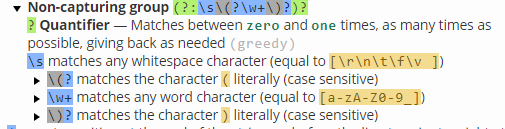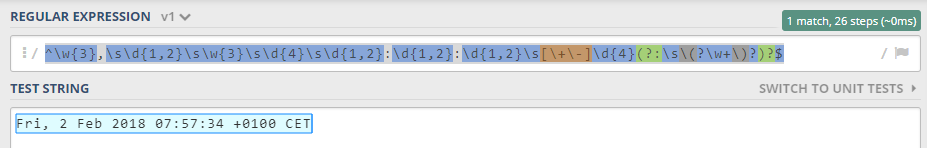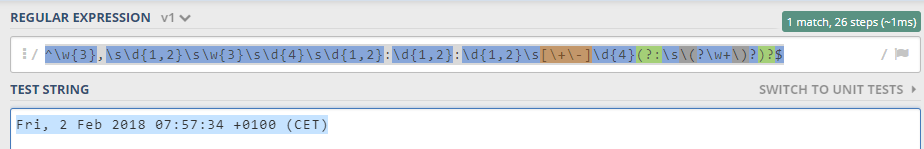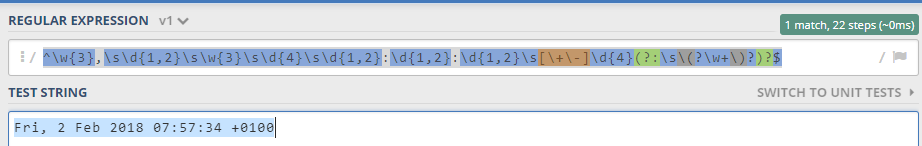To answer your last question. if you use the pattern below it will matche date with/without offset and with/without timezone. any combination of the date formats you listed will be matched.

^\w{3},\s\d{1,2}\s\w{3}\s\d{4}\s\d{1,2}:\d{1,2}:\d{1,2}(?:\s[\+\-]\d{4})?(?:\s\(?\w+\)?)?\$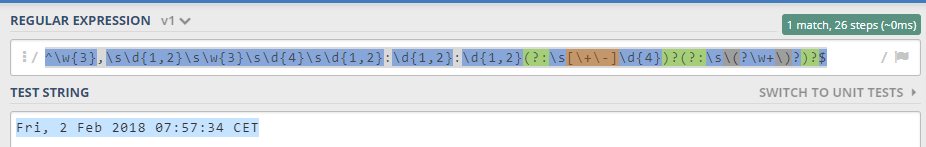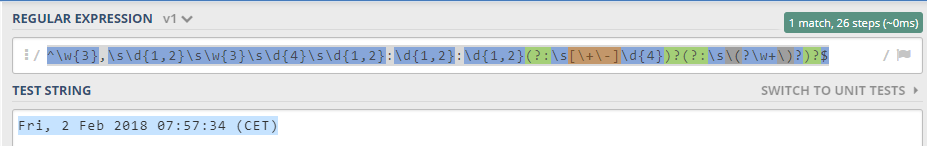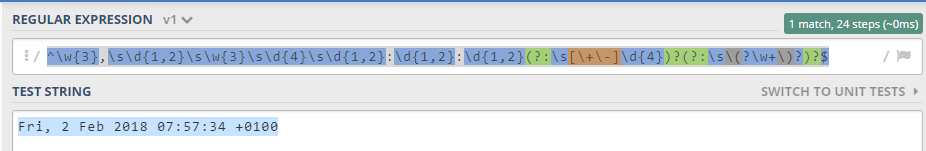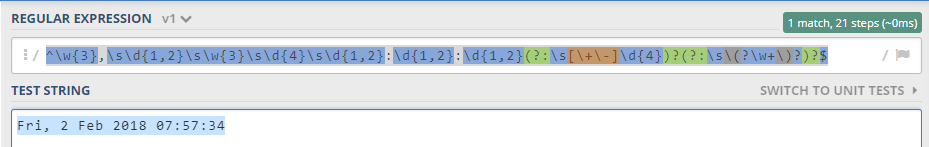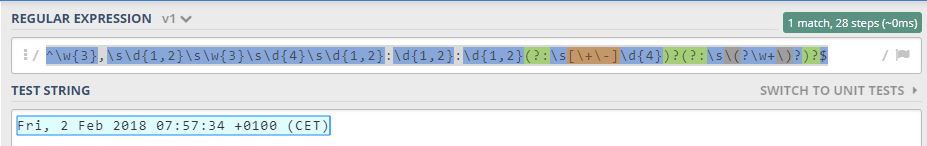Okay, they will be matched, but is there a way to scan for a pattern with a literal time zone? Suppose there's a format like this:

Tue, 2 Jan 2018 19:03:52 CET

What scan pattern to use so that it's properly converted to the following?

2018-01-02T18:03:52Z